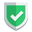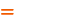我们相信：世界是美好的，你是我也是。平行空间的世界里面，不同版本的生活也在继续...

## 正常可以捕获

``````try:
s = 168 / 0
except Exception as err:
print(err)``````

``division by zero``

``````try:
n = 168 / 0.0
except Exception as err:
print(err)``````

``float division by zero``

## 无法捕获的情况

``````import numpy as np
a = np.array()
try:
sn = 168 / a
except Exception as err:
print(err)
finally:
print(a, type(a))``````

``````import numpy as np
y_true = np.array([0, 1])
y_pred = np.array([1, 1])
tn = sum((y_true == 0) & (y_pred == 0))
try:
p = 666 / tn
except Exception as err:
print(err)
finally:
print(tn, type(tn))``````

``RuntimeWarning: divide by zero encountered in long_scalars``

• 都涉及`numpy`，分母零的类型都不是普通的`int32`，是`numpy.int32`
• 提示信息的类型是`warning`警告，并不是`exception`异常，所以没有被捕获到。
• 代码并没有停止运行，继续执行了。即使分母为零，最后结果也返回值为零。

## 解决方案

``````import numpy as np
s = np.array([0, 1])
n = np.array([1, 1])
tn = sum((s == 0) & (n == 0))
t = 666/tn if (tn>0) else 0
print(t)``````

## 结束语如果本文对您有帮助，或者节约了您的时间，欢迎打赏瓶饮料，建立下友谊关系。本博客不欢迎：各种镜像采集行为。请尊重原创文章内容，转载请保留作者链接。【福利】 腾讯云最新爆款活动！1核2G云服务器首年50元！【源码】本文代码片段及相关软件，请点此获取更多信息【绝密】秘籍文章入口，仅传授于有缘之人python